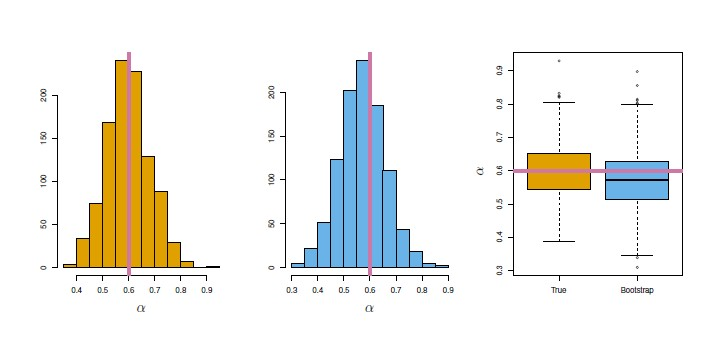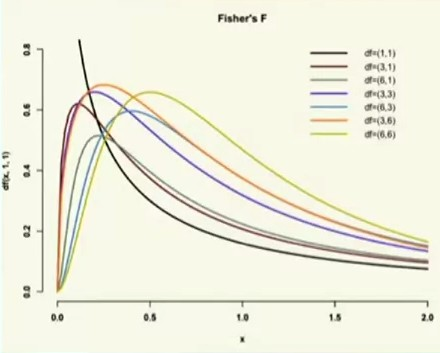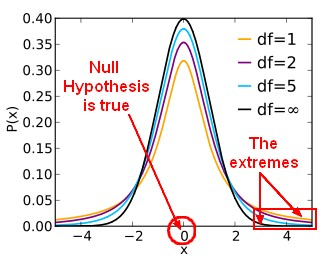# Statistics - Sampling Distribution

Distribution of estimated statistics from different samples (same size) from the same population is called a sampling distribution

This is called a sampling distribution not a sample distribution

It permits to make probability judgement about samples.

Because of the central limit theorem, sampling distributions are known to be normal and therefore are fundamental to inferential statistics because they allow for probabilistic predictions about outcomes.

## Demonstration

The code below showcase the fact that a sample distribution created from the mean of a lot of sample from the same population has a normal form.

• Creating the population data randomly distributed
``````population_n = 10000;
population_data = [];
population_max = 100;
population_data = [];

for (i = 0; i < population_n; i++) {
random_value = Math.floor(Math.random() * Math.floor(population_max));
population_data.push(random_value);
}

histogram({ selector: "population", data: population_data});```
```
• Sampling the population 1000 times with a sample size of 20, calculating the mean and adding it to the sample distribution
``````// Sample Data
sample_distribution_data = [];
sample_distribution_n = 1000;
for (j = 0; j < sample_distribution_n; j++) {
sample_data = [];
sample_n = 20;
for (i = 0; i < sample_n; i++) {
population_random_index = Math.floor(
Math.random() * Math.floor(population_max)
);
sample_data.push(population_data[population_random_index]);
}
sample_distribution_data.push(d3.mean(sample_data));
}
histogram({ selector: "sample", data:sample_distribution_data});```
```

## Documentation / Reference

Recommended PagesR - Bootstrap

in R. Bootstrap lets you get a look at the sampling distribution of statistics, for which it's really hard to develop theoretical versions. Bootstrap gives us a really easy way of doing statistics when...Statistics - (Estimator|Point Estimate) - Predicted (Score|Target|Outcome| )

An estimator or point estimate is a statistic that is used to infer the value of an unknown parameter in a statistical model. A point is a value in this entire possible range of values from the distribution....Statistics - (dependent|paired sample) t-test

A dependent t-test is appropriate when: we have the same people measured twice. the same subject are been compared (ex: Pre/Post Design) or two samples are matched at the level of individual subjects...Statistics - Bootstrap Resampling

Bootstrap is a powerful resampling method for assessing uncertainty in estimates and is particularly good for getting their: standard errors and confidence limits. Why is the bootstrap useful? The...Statistics - Central limit theorem (CLT)

The Central_limit_theoremcentral limit theorem (CLT) is a probability theorem (unofficial sovereign) It establishes that when: random variables (independent) (estimate of a random process) are added...Statistics - F-distributions

Like the t-test and family of t-distributions, the F-test has a family of F-distributions The family of F-distributions depends on: the Number of subjects per group the Number of groups ANOVA...Statistics - t-distributions

The t-distributions are used to calculate the p-value (basically you look it up in a table, nowadays software will compute it). It's basically a normal random variable except for small numbers of samples....